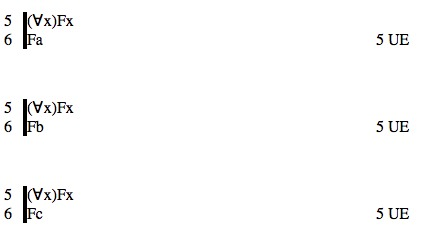# Tutorial 18: Universal Elimination

Logical System

2013

### Skill to be acquired:

To understand the concepts of scope, free, bound. To meet substitution and the rule for removing a Universal Quantifier.

Bergmann The Logic Book Section 10.1.

### The Tutorial

There is a new rule of derivation-- Universal Elimination (often called 'Universal Instantiation')-- which, roughly speaking, allows you to do the following... if a formula has a Universal Quantifier as its main connective you may remove the Universal Quantifier and have as a new line the body of the formula with a constant term substituted in for all free occurrences of the variable of quantification within the scope. For example,are all proof fragments illustrating Universal Elimination.

Notice the form of a formula with a quantifier as its main connective. Examples of such formulas are (∀x)(Fx), (∃x)(Fx&Gx), (∀x)(Fx⊃Gx). In each case there is the quantifier with its variable, enclosed within brackets, and this is followed by a formula known as the scope of the quantifier. In our examples, (Fx) is the scope of the first one, (Fx&Gx) the scope of the second, and (Fx⊃Gx) the scope of the third.

More formally...

If <scope> is any well-formed formula then (∀<variable>)<scope> is the universal quantification of <scope> using <variable> and (∃<variable>)<scope> is the existential quantification of <scope> using <variable> . In each case the <scope> is the scope of the quantification.

The notion of the scope of a quantifier is important; and it is good practice to enclose the scope in brackets so as to display exactly what it is. For example, the scope of the formula (∀x)Fx⊃Gx is Fx not (Fx⊃Gx); the formula as a whole will be read as ((∀x)(Fx))⊃Gx; such a formula is better written as (∀x)(Fx)⊃Gx.

A quantifier binds all occurrences of its <variable> in its scope. An occurrence of a variable which is not bound is called free. So, in (∀x)(Fx)⊃Gx the occurrence of x immediately after F is bound whereas the occurrence of x immediately after G is free; but in the formula (∀x)(Fx⊃Gx) both occurrences are bound.

Substitutions are on occasions made of one term for free occurrences of another-- in Universal Elimination, for example.The notation <wff>[<newterm>/<oldterm>] means the formula <wff> with <newterm> substituted for the free occurrences of <oldterm> throughout. So, for example, (Fx⊃Gx)[a/x] is (Fa⊃Ga).

#### The Rule of Universal Elimination UE

If a derivation contains a line of the form

n (∀<variable>)<scope> <any justification>

then a line of the form

<<scope>[<constant>/<variable>]> 'n UE'

may be added to the derivation. Remember, in this system, the constants are the lower case letters {a,b,c...,m}.

#### A note of relevance to more advanced work: terms, atomic and compound terms, constant or closed terms

In logic, the word 'term' roughly means name. And thus far we have met two kinds of term: the constants {a,b,c...,m} and the variables {n,o,p...,z}. The constants have been used to symbolize proper names like 'Arthur', 'Beryl' and so on. The variables have been used to construct the quantified formulas such as (∀x)Fx.

The rule Universal Elimination permits the dropping of a Universal Quantifier and moving to the scope with a constant substituted throughout.

However, more advanced logical systems permit more advanced kinds of terms. Consider the English phrase 'the father of Arthur'. This is a perfectly good name, and more advanced systems would allow it to be symbolized perhaps by 'f(a)'. In such systems, the ordinary constants {a,b,c...,m} and variables {n,o,p...,z} are considered to be atomic terms. And terms like 'f(a)', 'f(b)', 'f(f(a))' , etc. are compound or functional terms. Some compound terms have variables in them, eg 'f(x)' (which means 'the father of x') and others do not, eg 'f(a)'.

The terms, atomic or compound, which do not have variables in them are called closed terms.

The rule of Universal Elimination, in more advanced systems, actually permits the substitution of any closed term as an instantiation. For example, it permits

 5 (∀x)Fx 6 Ff(a) 5 UE

(which might represent a formal proof of the English inference 'Everything is friendly; therefore, The father of Arthur is friendly'.)

We will meet this in later, more advanced, Notes on functional terms and identity; these Notes are supplementary to this course.

## Exercise to accompany Predicate Tutorial 8

### Exercise 1 (of 3)

Identify the scopes of the following formulas

a) (∀x)(Fx)
b) (∀x)(Fx⊃Gx)
c) (∀x)(Fx)⊃Gx
d) (∃x)(Fx)&Gx
e) (∃x)(Fx&Gx)
f) (∀y)(Hy⊃(∃x)(Fx&Gx))
g) (∀y)((∀x)((∀z)(Hy&(Fz&Gx))))

a) (Fx)

b) (Fx⊃Gx)

c) This is a bit of a trick question, sorry. (∀x)(Fx)⊃Gx has as its main connective the ⊃ so the formula as a whole is not a quantified formula and does not have a scope. However, if our interest is with the quantified formula (∀x)(Fx) which is a subformula of (∀x)(Fx)⊃Gx then it has scope (Fx).

d) This also is a trick. (∃x)(Fx)&Gx has as its main connective the & so the formula as a whole is not a quantified formula and does not have a scope. However, if our interest is with the quantified formula (∃x)(Fx) which is a subformula of (∃x)(Fx)&Gx then it has scope (Fx).

e) (Fx&Gx)

f) (Hy⊃(∃x)(Fx&Gx))

g) ((∀x)((∀z)(Hy&(Fz&Gx))))

### Exercise 2 (of 3)

Identify the free and bound occurrences of the variable x in following formulas

a) (∀x)(Fx)
b) (Fx⊃Gx)
c) Fx⊃ (∀x)(Gx)
d) (∀x)(Fx)⊃Gx
e) (∃x)(Fx)&Gx
f) (∃x)(Fx&Gx)

a) (∀x)(Fxbound)

b) (Fxfree⊃Gxfree)

c) Fxfree⊃ (∀x)(Gxbound)

d) (∀x)(Fxbound)⊃Gxfree

e) (∃x)(Fxbound)&Gxfree

f) (∃x)(Fxbound&Gxbound)

### Exercise 3 (of 3)

Derive the following valid arguments

(a) (∀x) (Fx) ∴ Fa
(b) (∀x) (Fx) ∴ Fa&Fb
(c) Fc,(∀x) (Fx⊃Gx) ∴ Gc
(d) Fa,(∀x) (Fx⊃Gx), (∀x) (Gx⊃Hx) ∴Ha
(e) (∀x) (Fx⊃Gx), (∀x) (Gx⊃Hx) ∴(Fa⊃Ha)

If you decide to use the web application for the exercises you can launch it from here Deriver[Bergmann]  — username 'logic' password 'logic'. Then either copy and paste the above formulas into the Journal or use the Deriver File Menu to Open Web Page with this address https://softoption.us/test/easyDeriver/CombinedExercisesEasyDBergmann.html .

Preferences

You may need to set some Preferences for this.

• you can check that the parser is set to bergmann.